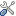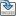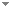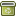# Mathematical electron model and the SI unit 2017 Special Adjustment

 Authors Abstract Following the 26th General Conference on Weights and Measures are fixed the numerical values of the 4 physical constants ($h, c, e, k_B$). This is premised on the independence of these constants. This article discusses a model of a mathematical electron from which can be defined the Planck units as geometrical objects (mass M=1, time T=2$\pi$ ...). In this model these objects are interrelated via this electron geometry such that once we have assigned values to 2 Planck units then we have fixed the values for all Planck units. As all constants can then be defined using geometrical forms (in terms of 2 fixed mathematical constants, 2 unit-specific scalars and a defined relationship between the units $kg, m, s, A$), the least precise CODATA 2014 constants ($G, h, e, m_e, k_B$...) can then be solved via the most precise ($c, \mu_0, \alpha, R_\infty$), with numerical precision limited by the precision of the fine structure constant $\alpha$. In terms of this model we now for example have 2 separate values for elementary charge, calculated from ($c, \alpha, R_\infty$) and the 2017 revision. Keywords CODATA  SI unit  2017 Special Adjustment  definition kg  electron  virtual electron  mathematical electron Categories (categorize this paper) OptionsEdit this recordMark as duplicateExport citationFind it on ScholarRequest removal from indexRevision history

PhilArchive copy

Setup an account with your affiliations in order to access resources via your University's proxy server
Configure custom proxy (use this if your affiliation does not provide a proxy)

## Citations of this work BETA

No citations found.

## Similar books and articles

The Limits of Special Relativity.B. G. Sidharth - 2008 - Foundations of Physics 38 (8):695-706.
When Can a Classical Electron Accelerate Without Radiating?Philip Pearle - 1978 - Foundations of Physics 8 (11-12):879-891.
A Model of the Electron in a 6-Dimensional Spacetime.Paolo Lanciani - 1999 - Foundations of Physics 29 (2):251-265.
Electron Spin or “Classically Non-Describable Two-Valuedness”.Domenico Giulini - 2008 - Studies in History and Philosophy of Science Part B: Studies in History and Philosophy of Modern Physics 39 (3):557-578.
A Theoretical Determination of the Electronic Rest Mass.J. P. Kobus - 1975 - Foundations of Physics 5 (2):343-347.
Fine-Structure Constant From Golden Ratio Geometry.Michael A. Sherbon - 2018 - International Journal of Mathematics and Physical Sciences Research 5 (2):89-100.